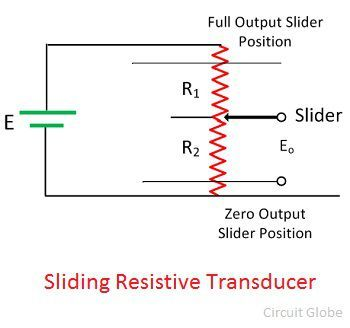# Resistive Transducer

Definition: The transducer whose resistance varies because of the environmental effects such type of transducer is known as the resistive transducer. The change in resistance is measured by the ac or dc measuring devices. The resistive transducer is used for measuring the physical quantities like temperature, displacement, vibration etc.

The measurement of the physical quantity is quite difficult. The resistive transducer converts the physical quantities into variable resistance which is easily measured by the meters. The process of variation in resistance is widely used in the industrial applications.

The resistive transducer can work both as the primary as well as the secondary transducer. The primary transducer changes the physical quantities into a mechanical signal, and secondary transducer directly transforms it into an electrical signal.

Example – The circuit of the sliding resistive transducer is shown in the figure below.  The sliding contacts are placed on the resistive element. The slider moves horizontally. The movement of the slider changes the value of the resistive element of the transducer which is measured by the voltage source E.The displacement of the slider is converted into an electrical signal.

The following are the advantages of the resistive transducer.

1. Both the AC and DC, current or voltage is appropriate for the measurement of variable resistance.
2. The resistive transducer gives the fast response.
3. It is available in various sizes and having a high range of resistance.

## Working Principle of Resistive Transducer

The resistive transducer element works on the principle that the resistance of the element is directly proportional to the length of the conductor and inversely proportional to the area of the conductor.Where R – resistance in ohms.
A – cross-section area of the conductor in meter square.
L – Length of the conductor in meter square.
ρ – the resistivity of the conductor in materials in ohm meter.

The resistive transducer is designed by considering the variation of the length, area and resistivity of the metal.

### Applications of Resistive Transducer

The following are the applications of the resistive transducer.

1. Potentiometer  – The translation and rotatory potentiometer are the examples of the resistive transducers. The resistance of their conductor varies with the variation in their lengths which is used for the measurement of displacement.
2. Strain gauges –  The resistance of their semiconductor material changes when the strain occurs on it. This property of metals is used for the measurement of the pressure, force-displacement etc.
3. Resistance Thermometer  – The resistance of the metals changes because of changes in temperature. This property of conductor is used for measuring the temperature.
4. Thermistor – It works on the principle that the temperature coefficient of the thermistor material varies with the temperature. The thermistor has the negative temperature coefficient. The Negative temperature coefficient means the temperature is inversely proportional to resistance.

There are a number of ways because of which the resistance of the metal changes with the changed in the physical phenomenon. And this property of conductors is used for measuring the physical quantities of material.